# 微量钛调控金刚石/铜复合材料界面及热性能Enhancing the Interface Bonds and Thermal Properties of Diamond/Copper Composites via Micro-Titanium

• 全文下载: PDF(2443KB)    PP.1135-1145   DOI: 10.12677/MS.2018.812136
• 下载量: 210  浏览量: 376   科研立项经费支持

The addition of titanium element into Copper matrix for the alloying was confirmed to improve the interface bonds of Copper and diamond composites. In the sintering processing the effect of the optimized fraction volume of diamond and titanium elements on microstructure, thermal and interface bond of the composites was investigated. The study results indicate that the interface adhesion force of the composites is extremely improved by controlling the fraction volume of di-amond and titanium. The thermal conductivity of fabricated diamond/copper composites with the 3 vol.% titanium elements reaches 670 W/(m•K) at 1080˚C for 5 h, achieving the predicted value of 90% due to the formation of TiC and Cu3Ti2 on the diamond/copper interface obtaining the better interfacial bonding to make the composites attain the higher thermal conductivities. In addition, the surface stress dynamics of the gaps between diamonds filled with liquid metal at high temperatures is calculated in this study.

1. 引言

2. 实验及方法

$\lambda =D\rho C$ (1)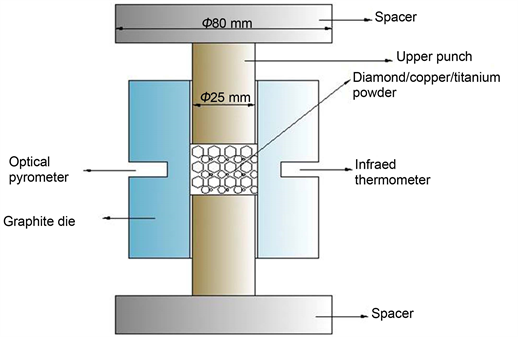Figure 1. The diagram of die sinteringTable 2. Relative density of samples with the different volume fraction of DiamondTable 3. Relative density of samples with the different volume fraction of titanium and 45 vol.% DiamondTable 4. Relative density of samples with the different volume fraction of titanium and 50 vol.% Diamond

3. 结果与讨论

3.1. 相的组成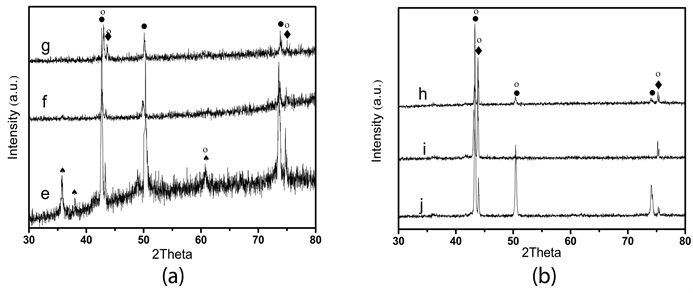Figure 2. The XRD spectrum of diamond/copper composites with the different volume fraction of diamond (a) 45 vol.% and (b) 50 vol.%. Legend: ●-Copper, ♦-Diamond, ♠-TiC, ○-Cu3Ti2

$\text{Ti}\left(\text{S}\right)+\text{C}\left(\text{S}\right)=\text{TiC}\left(\text{S}\right)\text{\hspace{0.17em}}\text{\hspace{0.17em}}\text{\hspace{0.17em}}\text{\hspace{0.17em}}\text{\hspace{0.17em}}\Delta G=\left(-186+0.0132T\right)\text{\hspace{0.17em}}\text{kJ}\cdot {\text{mol}}^{-\text{1}}$ (2)

${\text{Cu}}_{\text{3}}{\text{Ti}}_{\text{2}}+\text{2C}=\text{2TiC}+\text{3Cu}$ (3)

3.2. 微观结构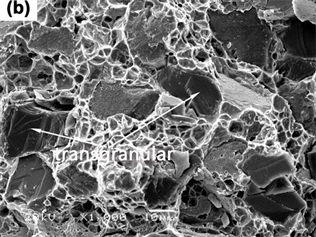Figure 3. The fracture morphologies of diamond/copper

45 vol.%金刚石/铜复合材料的显微组织结构如图5所示，扫面电镜观察到在铜基体和金刚石界面处发生塑性断裂，界面处的晶体结构组成为金刚石/碳化钛/金属间化合物的结构，这种结构大大改善了界面粘结性如由图5中的箭头所示。铜/金属间化合物/TiC附着在金刚石表面上，样品e的结果展示其界面结合强度大于金刚石颗粒的结合强度，这是由于微量钛元素的加入大大地改善了金刚石/铜界面的晶体结构组成形成。考虑到热学性质和复合材料的显微结构相关，因此，在本文中我们优化参数钛3 vol.%和45 vol.%金刚石能够获得良好的结合界面。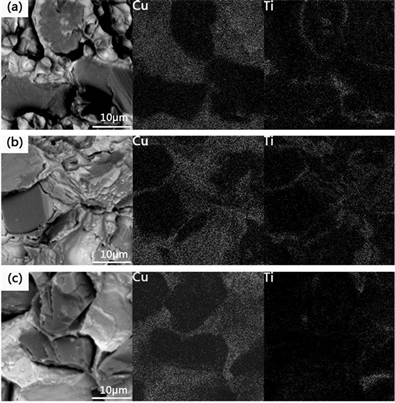Figure 4. The fracture microstructures 45 vol.% diamond/copper composites and the distribution of copper and titanium elements on the fracture surface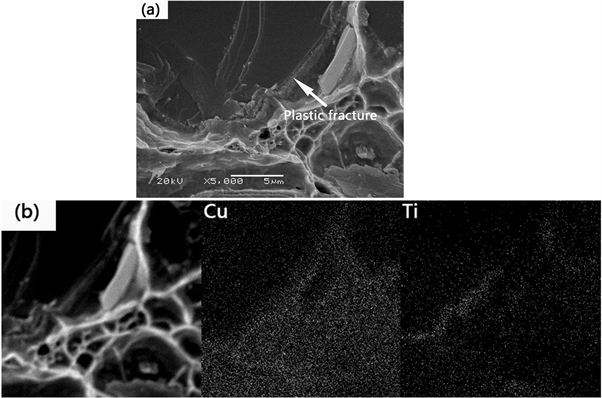Figure 5. The fracture microstructures 45 vol.% diamond/copper composites and the distribution of copper and titanium elements on the fracture surface

3.3. 液态金属表面张力动力学计算

${R}_{e}=\frac{\rho \upsilon d}{\mu }$ (4)

$d=f\cdot R$ (5)

$R=\frac{{V}_{p}}{{S}_{p}}=\frac{1}{{S}_{v}}$ (6)

${S}_{v}=\sqrt{\frac{{C}_{0}f\left(\varphi \right)}{k}}$ (7)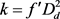(8)

$d=\frac{f{D}_{d}\left(1-\varphi \right)}{\varphi }\sqrt{\frac{{f}^{\prime }}{{C}_{0}\varphi }}$ (9)

${f}^{\prime }$ 表示形状因子，C0是一个常量，对于金刚石， ${f}^{\prime }$ 和C0的值分别为6.54 × 10−4和0.2，因此，式子(9)可以表示为式子(10)。

$d=1.144{D}_{d}\left(1-\varphi \right){\varphi }^{-3/2}$ (10)

 (11)

Vs和Vp分别表示固态颗粒和孔的体积分数，液流和固态的接触面积常数As，设想所有的孔都是开孔，可以用下式(12)表示：

$\left(\frac{{V}_{p}}{{A}_{s}}\right)=\frac{V{\varphi }_{s}}{{A}_{s}\left(1-\varphi \right)}$ (12)

${d}_{s}=\frac{4{V}_{s}\varphi }{{A}_{s}\left(1-\varphi \right)}$ (13)

$\Delta p=\frac{128\mu {L}_{e}Q}{\text{π}{R}^{4}}$ (14)

$\Delta p$ 为压力梯度， $Q=vA=v\text{π}{R}^{2}=v\text{π}{d}^{2}/4$ 。代替实际流速 $\left(v/\varphi \right)\left({L}_{e}/L\right)$ ，式子(13) (14)可以写成下式：

$\Delta p=\frac{128\mu {L}_{e}Q}{\text{π}{R}^{4}}\frac{\text{π}{d}^{2}}{4}\nu =\frac{32\mu {L}_{e}}{{d}^{2}}\frac{\nu }{\varphi }\frac{{L}_{e}}{L}$ (15)

$\nu =\frac{\Delta p{d}^{2}\varphi L}{32\mu {L}_{e}^{2}}=\frac{\Delta p}{L}\frac{{\varphi }^{3}}{1-\varphi }\frac{1}{\mu \beta {\left({v}_{s}/{A}_{s}\right)}^{2}}$ (16)

$\beta =2\left({L}_{e}/L\right)$ 值是一个Kozeny’s常数，代表的是中等空隙的变化在3.5~6.0之间，式子(16)表明孔隙度 $\varphi$ 对流速v影响是重要的，孔隙度的变化影响流速的变化。把金刚石看成球形其半径为90 mm，Kozeny常数β = 4.75，m = 3.41 × 10−3 Ns∙m−2，那么 ${V}_{s}/{A}_{s}=\left(4\text{π}{R}_{3}/3\right)/\left(4/{r}^{2}\right)=R/3=1.75×{10}^{-5}\text{\hspace{0.17em}}{\text{m}}^{-1}$ ，因此式子(16)可以写成下式：

$\nu =\frac{2×{10}^{11}\Delta p}{L}\frac{{\varphi }^{3}}{{\left(1-\varphi \right)}^{2}}$ (17)

${p}_{c}=\frac{2{\gamma }_{{l}_{g}}\mathrm{cos}\theta }{R}$ (18)

${p}_{\mu }=-\frac{8\mu }{{R}^{2}}\nu x$ (19)

${\gamma }_{{l}_{g}}$ 为表面张力， $\theta$ 为接触角，用H + x代替H0，在单方向上液态金属总的流动压力为：

$\begin{array}{c}p={p}_{0}+{p}_{c}+{p}_{m}+{p}_{\mu }={p}_{0}+\frac{2{\gamma }_{{l}_{g}}\mathrm{cos}\theta }{r}+\rho g\left(H+x\right)-\frac{8\mu }{{r}^{2}}\nu x\\ ={p}_{0}+\frac{2{\gamma }_{{l}_{g}}\mathrm{cos}\theta }{r}+\rho g{H}_{0}-\frac{8\mu }{{r}^{2}}\nu x\end{array}$ (20)

R表示毛细半径，H和H0流速的长度或者高度， $\rho$ 表示液流的密度，可以得到式子(20)，如果外加压力P0和流速v为常数，样品的厚度成为重要的影响因素，当接触角 < 90˚，毛细压力有助于液态金属渗进空隙，对于铜/金刚石复合材料来说，当温度为1080˚C，P0 = 5.6 × 10−1 Pa，v：1.818 × 10−2 m∙s−1，r：8.458 × 103 kg∙m−3，θ: 18˚，H0：3 mm，R：mm和 ${\gamma }_{{l}_{g}}$ ：1.25 Nm−2 ( ${\gamma }_{{l}_{g}}=1355-0.20\left(T-1356\right)$ )。

3.4. 热导率

$\lambda =\left(\frac{2\left(\frac{{\lambda }_{m}}{{\lambda }_{d}}-\frac{{\lambda }_{m}}{a{h}_{c}}-1\right){V}_{m}+\frac{{\lambda }_{m}}{{\lambda }_{d}}+\frac{2{\lambda }_{m}}{a{h}_{c}}+2}{\left(1-\frac{{\lambda }_{m}}{{\lambda }_{d}}+\frac{{\lambda }_{m}}{a{h}_{c}}\right){V}_{m}+\frac{{\lambda }_{m}}{{\lambda }_{d}}+\frac{2{\lambda }_{m}}{a{h}_{c}}+2}\right){\lambda }_{m}$ (21)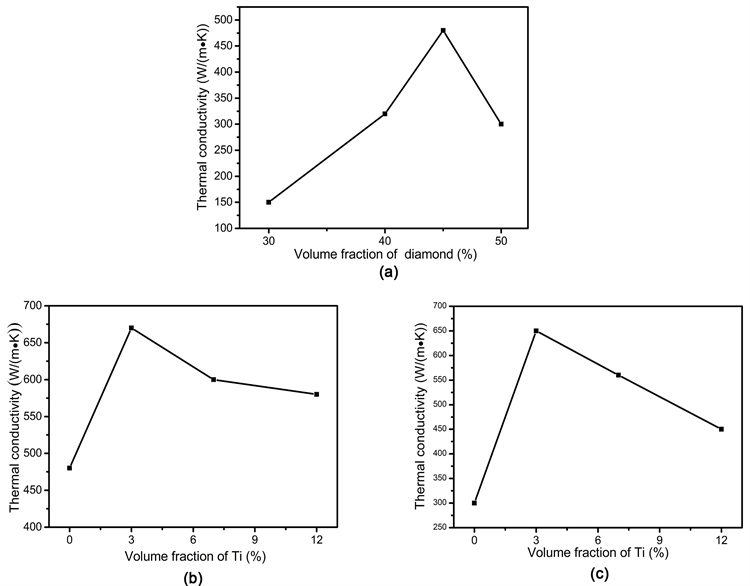Figure 6. The thermal conductivity of diamond/copper compositesTable 5. The specific heat capacity and heat diffusion coefficient of the measured composites

$\lambda =\left(\frac{2\left(\frac{{\lambda }_{m}}{{\lambda }_{d}}-1\right){V}_{m}+\frac{{\lambda }_{m}}{{\lambda }_{d}}+2}{\left(1-\frac{{\lambda }_{m}}{{\lambda }_{d}}\right){V}_{m}+\frac{{\lambda }_{m}}{{\lambda }_{d}}+2}\right){\lambda }_{m}$ (22)

4. 结论

NOTES

*通讯作者。

  He, H., Fu, R.L. and Shen, Y. (2007) Preparation and Properties of Si3N4/PS Composites Used for Electronicpackaging. Composites Science and Technology, 67, 2493-2499.  黄强, 顾明元. 电子封装材料的现状及发展[J]. 电子与封装, 2003, 3(2): 22-25.  童震松, 沈卓申. 金属封装材料的现状及发展[J]. 电子与封装, 2005, 5(3): 6-14.  邓安强, 樊静波, 谭占秋, 范根莲, 李志强, 张荻. 金刚石/铜复合材料在电子封装领域的研究[J]. 金刚石与磨料磨具工程, 2010, 5(30): 56-61.  Carl, Z. (2006) Thermal Materials Solve Power Electronics Challenges. Power Electronics Technology, 2, 40.  Stubblefied, M., Pang, S.S. and Cundy, V.A. (1996) Heat Loss in Insulated Pipe the Influence of Thermal Contact Resistance. A Case Study. Composites Part B: Engineering, 27, 85-93. https://doi.org/10.1016/1359-8368(95)00028-3  Wang, S. and Qiu, J. (2010) Enhancing Thermal Conductivity of Glass Fiber/Polymer Composites through Carbon Nanotubes Incorporation. Composites Part B: Engineering, 41, 533-536. https://doi.org/10.1016/j.compositesb.2010.07.002  Schubert, T., Trindade, B. and Weißgärber, T. (2008) Interfacial Design of Cu-Based Composites Prepared by Powder Metallurgy for Heat Sink Applications. Materials Science and Engineering: A, 475, 39-44. https://doi.org/10.1016/j.msea.2006.12.146  Cardarelli, F. (2008) Materials Handbook. Springer, England, London, 160-162, 173.  Katsuhito, Y. and Hideaki, M. (2004) Thermal Properties of Diamond/Copper Composite Material. Microelectronics Reliability, 44, 303-308. https://doi.org/10.1016/S0026-2714(03)00215-4  Xiao, Y.S., Xin, B.H., Shu, B.R., Hao, M.Z. and Xuan, H.Q. (2012) Effect of Molybdenum as Interfacial Element on the Thermal Conductivity of Diamond/Cu Composites. Journal of Alloys and Compounds, 529, 134-139. https://doi.org/10.1016/j.jallcom.2012.03.045  Andrey, M.A., Sergey, V.K. and Fedor, M.S. (2010) High Thermal Conductivity Composite of Diamond Particles with Tungsten Coating in a Copper Matrix for Heat Sink Ap-plication. Applied Thermal Engineering, 30, 1277-1291.  Hell, J., Chirtoc, C., Eisenmenger-Sittner, C., Hutter, H., Kornfeind, N., Kijamnajsuk, P., Kitzmantel, M., Neubauer, K. and Zellhofer, K. (2012) Characterisation of Sputter Deposited Niobium and Boron Interlayer in the Copper-Diamond System. Surface and Coatings Technology, 208, 24-31. https://doi.org/10.1016/j.surfcoat.2012.07.068  Xia, Y., Song, Y.Q., Lin, C.G., Cui, S. and Fang, Z.Z. (2000) Effect of Carbide Formers on Microstructure and Thermal Conductivity of Diamond-Cu Composites for Heat Sink Materials. Transactions of Nonferrous Metals Society of China, 19, 1161-1166.  Ekimov, E.A., Uetin, N.V.S., Popovich, A.F., et al. (2008) Thermal Conductivity of Diamond Composites Sintered under High Pressures. Diamond & Related Materials, 17, 838-843.  Chu, K., Liu, Z., Jia, C., et al. (2007) Thermal Conductivity of SPS Consolidated Cu/Diamond Composites with Cr-Coated Diamond Particles. Computational Materials Science, 41, 156-163.  Chu, K., Jia, C., Tian, W., Hui, X.L. and Guo, C.H. (2010) Thermal Conductivity of Spark Plasma Sintering Consolidated SiC/Al Composites Pores (Numerical Study and Experimental Validation). Composites Part A: Applied Science and Manufacturing, 41, 161-167. https://doi.org/10.1016/j.compositesa.2009.10.001  Chase Jr., M.W., Davies, C.A., Downey Jr., J.R. and Frurip, D.J. (1982) JANAF Thermochemical Tables. 4th Edition, Journal of Physical and Chemical Reference Data, No. 14, 1674.  Guo, W., Zhu, Y., Wang, L., Qu, P., Kang, H. and Chu, P.K. (2013) Microstructure Evolution and Mechanical Properties of Vacuum-Brazed C/C Composite with AgCuTi Foil. Materials Science and Engineering: A, 564, 192-198. https://doi.org/10.1016/j.msea.2012.11.057  Murray, J.L. (1987) Cu-Ti Phase Diagrams. In: Baker, H., Ed., Alloy Phase Diagrams, ASM International, Metals Park, 180.  Maxwell, J.C. (1904) A Treatise on Electricity and Magnetism. 3rd Edition, Oxford University Press, Oxford.  Hasselman, D.P.H. and Johnson, L.F. (1987) Effective Thermal Conductivity of Composites with Interfacial Thermal Barrier Resistance. Journal of Composite Materials, 21, 508-515. https://doi.org/10.1177/002199838702100602  Kiyoshi, M., Kanryu, I. and Yasuyuki, A. (2012) Processing of Diamond-Particles-Dispersed Silver-Matrix Composites in Solid-Liquid Co-Existent State by SPS and Their Thermal Conductivity. Composites Part B, 43, 1445-1452. https://doi.org/10.1016/j.compositesb.2011.08.003  Shu, B.R., Xiao, Y.S., Cai, Y.G., Nan, L., Jian, B.Z., Xin, B.H. and Xuan, H.Q. (2011) Effect of Coating on the Microstructure and Thermal Conductivities of Diamond-Cu Composites Prepared by Powder Metallurgy. Composites Science and Technology, 71, 1550-1555. https://doi.org/10.1016/j.compscitech.2011.06.012